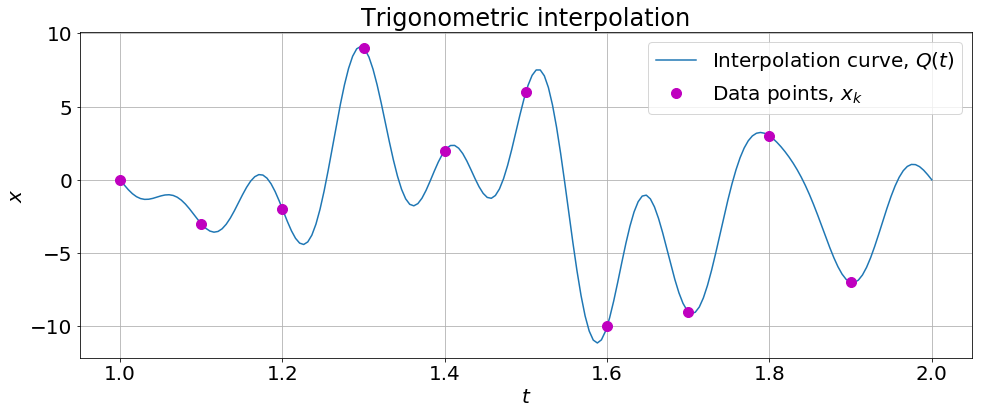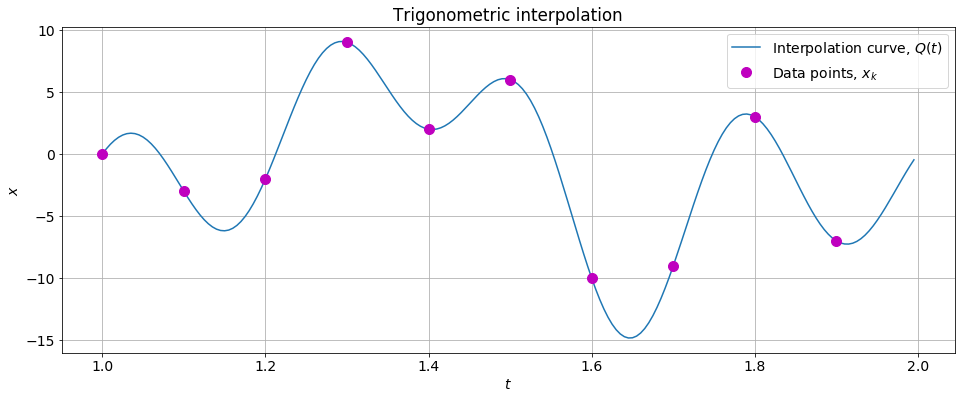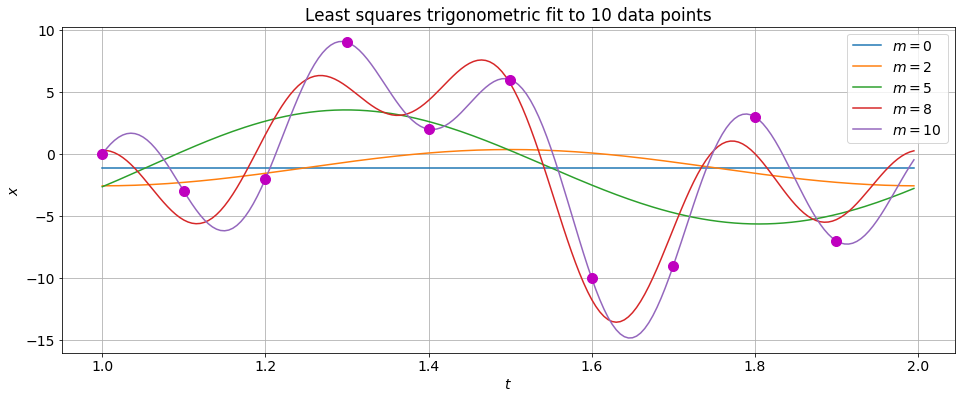# Trigonometric Interpolation¶

### Modules - Curve Fitting¶

Last edited: January 26th 2018

### Introduction¶

A trigonometric polynomial of degree $K$ can be written as a sum of sines and cosines of given periods

$$P_K(t)=a_0 + \sum_{k=1}^Ka_k\cos(kt)+\sum_{k=1}^Kb_k\sin(kt).$$

This form of interpolation is especially suited for periodic functions. The goal of trigonometric interpolation is to compute the $2n+1$ coefficients $a_0,a_1,...,a_K,b_1,b_2,...,b_K$, such that the function $P_K(t)$ passes through a given set of data points. We assume here that the data points are equally spaced. One way of solving this problem is to perform a polynomial interpolation on the unit circle in the complex plane, i.e. performing polynomial interpolation on

$$P(t)=\sum c_kz^t,$$

where $c_k=a_k+ib_k$ and $z=e^{it}$. This approach is used in . Thus, the discussion regarding polynomial interpolation can to some extent be covered using trigonometric interpolation. However, the trigonometric interpolating function $P(t)$ is obviously not unique, since $e^{it} = e^{i(t + 2\pi)} = e^{i(t + 4\pi)} =$ ..., thus requiring further manipulation.

For a discussion on polynomial interpolation (Lagrange, Chebyshev, Newton's divided difference, Runge's phenomenon, etc.), we suggest a look at our notebook Polynomial Interpolation. Here, we approach the solution using Discrete Fourier Transforms (DFTs) as in . Hence, some basic knowledge of DFTs will be helpful, which e.g. can be reviewed in another notebook titled Discrete Fourier Transform and Fast Fourier Transform.

OK, then. As always, we start by importing necessary libraries, and set common figure parameters.

In :
# Import libraries
import numpy as np
import matplotlib.pyplot as plt
import scipy.fftpack as fft
%matplotlib inline

# Casting unitary numbers to real numbers will give errors
# because of numerical rounding errors. We therefore disable
# warning messages.
import warnings
warnings.filterwarnings('ignore')

# Set common figure parameters
newparams = {'figure.figsize': (16, 6), 'axes.grid': True,
'lines.linewidth': 1.5, 'lines.markersize': 10,
'font.size': 14}
plt.rcParams.update(newparams)


### Generalize interpolation¶

Assume a given set of $n$ data points $(t_i,x_i)$ where $i=0,1,...,n-1$, and let $f_0(t), f_1(t),...,f_{n-1}(t)$ be given functions of $t$. We now require that the $n\times n$ matrix

$$A=\begin{bmatrix} f_0(t_0)&\cdots&f_0(t_{n-1})\\ \vdots&\ddots&\vdots\\ f_{n-1}(t_0)&\cdots&f_{n-1}(t_{n-1}) \end{bmatrix},$$

is unitary. That is, $A^{-1}=\overline{A}^T$. If $A$ is real, then $A^{-1}=A^T$ and the matrix is orthogonal. We now define $\vec y=A\vec x$, which implies that $\vec x=\overline A^T \vec y$. Written in terms of sums we then have $x_j=\sum_{k=0}^{n-1}y_k\overline{f_k(t_j)}.$ Thus, the function

$$F(t)=\sum_{k=0}^{n-1}y_k\overline{f_k(t)},$$

interpolates the given data set! If $A$ is a real $n\times n$ orthogonal matrix, then

$$F(t)=\sum_{k=0}^{n-1}y_kf_k(t).$$

Since the DFT can be written as a unitary matrix $F_n$, we can use this to interpolate a given data set.

### From discrete Fourier transform to trigonometric interpolation¶

From the previously mentioned notebook on DFTs, we have an excellent algorithm, the Fast Fourier Transform (FFT) algorithm for calculating

$$x_j = \frac{1}{n}\sum_{k=0}^{n-1}y_ke^{i2\pi kj/n},$$

which easily can be rewritten as

$$x_j=\sum_{k=0}^{n-1}y_k\frac{\exp\left[\frac{i2\pi k(t_j-c)}{d-c}\right]}{n},$$

where $t_j=c+j(d-c)/n$ for $j = 0, 1, ..., n-1$ for a given interval $[c,d]$. Assume that $\vec x = (x_0,x_1,...,x_{n-1})$ is a known set of data points. Then the complex function

$$Q(t)=\frac{1}{n}\sum_{k=0}^{n-1}y_k\exp\left[\frac{i2\pi k(t-c)}{d-c}\right]$$

satisfies $Q(t_j)=x_j$ for $j=0,1,...,n-1$. In other words, $Q(t)$ interpolates the set $\{(x_j,t_j); \,j=0,1,...,n-1\}$, and the interpolation coefficients are found by the Fourier transform! Note that $t_i$ has to be equally spaced. This is generally not required, but it simplifies matters.

In :
def trigInterp(x, c=0, d=1, N=100):
"""Calculates a trigonometric polynomial of degree n-1 that interpolates
a set of n complex (or real) data points. The data points can be written
as (t_i,x_i), i=0,1,2,..., where t_i are equally spaced on the interval
[c,d].

:x: complex or float numpy array. Data points.
:c: float. Start value t-axis. t.
:d: float. End value t-axis. t[N-1].
:N: int. Number of function evaluations of the interpolating function.
:returns: complex64 numpy array. Second axis of the interpolating function.
"""
t = np.linspace(c, d, N)  # t-values, first axis
n = len(x)  # Number of data points
y = fft.fft(x)  # Interpolating coefficients

# Evaluate sum
Q = np.zeros(N, np.complex64)
for k in range(0, n):
Q = Q + y[k]*np.exp(2j*np.pi*k*(t-c)/(d-c))

return Q/n


Let's try with an example. For simplicity, we let the data points $\vec x$ be real, although the above function can be used for complex data points as well.

In :
x = np.random.randint(-10, 10, 10)  # Data points
c, d = 1, 2  # Interval for t
N = 200  # Number of function evaluations

# Calculate interpolation curve
Q = trigInterp(x, c, d, N)

# Plot results
plt.figure()
plt.plot(np.linspace(c, d, len(Q)), Q, label=r'Interpolation curve, $Q(t)$')
plt.plot(np.linspace(c, d, len(x), False), x, 'mo', label='Data points, $x_k$')
plt.xlabel('$t$'), plt.ylabel('$x$'), plt.title('Trigonometric interpolation')
plt.legend();### Improve the algorithm – exploit periodicity and remove high frequencies¶

Using Euler's formula we can write $Q(t)=P(t)+iI(t)$. In the following discussions we are going to assume that the elements $x_j$ are real, such that $Q(t)=P(t)$, and let $y_k=a_k+ib_k$. The following discussion can be applied to $I(t)$ as well. From the definition of the DFT, it is clear that $y_0$ has to be real and $y_{n-k} = \overline{ y_k}$ if every element in $x$ are real. Moreover, we can exploit the periodicity $\cos(2\pi n-r)=\cos(r)$ and $\sin(2\pi n-r)=-\sin(r)$ to make a more effective algorithm for calculating the interpolation curve. It should by now be clear that the trigonometric interpolating polynomial is not unique, as explained in the introduction.

The interpolation curve becomes

$$Q(t)=P_n(t)=\frac{1}{n}\sum_{k=0}^{n-1}\left(a_k \cos\frac{2\pi k(t-c)}{d-c}-b_k \sin\frac{2\pi k(t-c)}{d-c}\right).$$

If $n$ is even, we can simlify this as

$$P_{n,\text{even}}(t)=\frac{a_0}{n}+\frac{2}{n}\sum_{k=1}^{n/2-1}\left(a_k \cos\frac{2\pi k(t-c)}{d-c}-b_k \sin\frac{2\pi k(t-c)}{d-c}\right)+\frac{a_{n/2}}{\sqrt n}\cos \frac{n\pi(t-c)}{d-c},$$

thus halving the number of calculations needed. Note that this interpolating polynomial is not the same as in the previous section. All the shorter periods are not included, and thus the result will be somewhat smoother. If $n$ is odd the interpolation curve becomes

$$P_{n,\text{odd}}(t)=\frac{a_0}{n}+\frac{2}{n}\sum_{k=1}^{(n-1)/2}\left(a_k \cos\frac{2\pi k(t-c)}{d-c}-b_k \sin\frac{2\pi k(t-c)}{d-c}\right).$$

These expressions can be evaluated and plotted as before. We can however do better. We are going to view the coefficients of $P(t)$ as coefficients of an $N\geq n$ order trigonometric polynomial. In other words, $P_n(t)=P_N(t)$, where we set $a_k=b_k=0$ for $k=n/2+1,n/2+1,...,N/2$ and $a_k+ib_k=y_k$ for $k=0,1,...,n/2$. If we perform an inverse Fourier transform of $\frac{N}{n}P_N(t)$, we get $N$ data points lying on $P(t)$! Let's do that and see how it performs.

In :
def fastTrigInterp(x,c,d,N=100):
""" Calculates a trigonometric polynomial of degree n/2 (n even) or
(n-1)/2 (n odd) that interpolates a set of n real data points. The
data points can be written as (t_i,x_i), i=0,1,2,..., where t_i are
equally spaced on the interval [c,d].

:x: complex or float numpy array. Data points.
:c: float. Start value t-axis. t.
:d: float. End value t-axis. t[N-1].
:N: int. Number of function evaluations of the interpolating function.
:returns: float numpy array. Second axis of the interpolating function.
"""
n = len(x)  # Number of data points
y = fft.fft(x)  # Interpolating coefficients

# Interpolating coefficients as viewed as a
# trigonometric polynomial of order N.
yp = np.zeros(N, np.complex64)
yp[0:int(n/2)] = y[0:int(n/2)]
yp[N - int(n/2):N] = y[int(n/2):n]

return np.real(fft.ifft(yp))*N/n


Let's try out our new interpolation function on the same data set as above.

In :
# Calculate interpolation curve
xp = fastTrigInterp(x, c, d, N)

# Plot results
plt.figure()
plt.plot(np.linspace(c, d, N, False), xp, label=r'Interpolation curve, $Q(t)$')
plt.plot(np.linspace(c, d, len(x), False), x, 'mo', label='Data points, $x_k$')
plt.xlabel('$t$'), plt.ylabel('$x$'), plt.title('Trigonometric interpolation')
plt.legend();By exploiting the periodicity of the data points and excluding the higher frequencies we clearly see that the interpolation curve $Q(t)$ is smoother, and hence fits the data points "better" than our previous $Q(t)$ curve.

### Least squares fitting¶

When the number of data points becomes large, it is unusual to fit a model function exactly. Then, we are often instead interested in fitting an approximated curve. This is often done when analyzing measurements.

The least squares problem  for this setup can be formulated as follows. Given a linear system $A_m\vec x=\vec y$ of $m$ equations, find a vector $\vec x$ that minimizes $||\vec y-A\vec x||$. In other words, we have an inconsistent system of $m$ equations that we want to solve "as best as possible". It can be shown that for every linear system $A_m\vec x=\vec y$, the corresponding normal system $A_m^TA_m\vec x = A_m^T \vec y$ is consistent. Moreover, all solutions of the normal system are least squares solutions of $A_m\vec x=\vec y$.

Let's apply this to the generalized interpolation above. $A_m$ is then the matrix of the first $m$ rows of $A$. Since $A$ is unitary (and orthogonal if $A$ is real), the column vectors and row vectors of $A$ form orthonormal sets. Likewise, the column vectors and row vectors of $A_m$ will form orthonormal sets. Thus, the normal equation becomes

$$A_m^TA_m\vec x = I\vec x = \vec x = A_m^T \vec y.$$

This means that the least squares solution for $F_n(t)=\sum_{k=0}^{n-1}y_kf_k(t)$ using only the first $m$ equations is

$$F_m(t)=\sum_{k=0}^{m-1}y_kf_k(t).$$

For the case of trigonometric interpolation, this means that the least squares solution of degree $m$ is simply done by filtering out the $n-m$ terms of the highest frequencies. These arguments hold for both functions trigInterp and fastTrigInterp above. We are now going to create a function calculating the least squares solution, based on the latter.

In :
def leastSquaresTrig(x, m, c=0, d=1, N=100):
"""Calculates a least squares trigonometric polynomial fit of degree
about m/2 of n real data points. The data points can be written as
(t_i,x_i), i=0,1,2,..., where t_i are equally spaced on the interval
[c,d].

:x: complex or float numpy array. Data points.
:c: float. Start value t-axis. t.
:d: float. End value t-axis. t[N-1].
:N: int. Number of function evaluations of the fitted function.
:returns: float numpy array. Second axis of the fitted function.
"""
n = len(x)  # Number of data points
if not 0<=m<=n<=N:
raise ValueError('Is 0<=m<=n<=N??')
y = fft.fft(x)  # Interpolating coefficients

# Interpolating coefficients viewed as a
# trigonometric polynomial of order N.
yp = np.zeros(N, np.complex64)

# Use only m lowest frequencies
yp[0:int(m/2)] = y[0:int(m/2)]
yp[N - int(m/2) + 1:N] = y[n - int(m/2) + 1:n]

# If odd m, terms corresponding to m/2 has both a sine
# and a cosine term
if (m % 2):
yp[int(m/2)] = y[int(m/2)]
# If even m, terms corresponding to m/2 has only a cosine term
else:
yp[int(m/2)] = np.real(y[int(m/2)])
if m<n and m>0:
yp[N - int(m/2)] = yp[int(m/2)]

return np.real(fft.ifft(yp))*N/n


We now perform trigonometric interpolation on the same random data points as earlier, excluding an increasing number of frequencies. As we have seen, this implies that we are finding the least squares solutions for a trigonometric polynomial of decreasing degree.

In :
plt.figure()
for m in [0, 2, 5, 8, 10]:
# Calculate interpolation curve
xp = leastSquaresTrig(x, m, c, d, N)
# Plot results
plt.plot(np.linspace(c, d, len(xp), False), xp, label=r'$m=%d$' % m)

plt.plot(np.linspace(c, d, len(x), False), x, 'mo')
plt.xlabel('$t$'), plt.ylabel('$x$')
plt.title('Least squares trigonometric fit to %d data points' % len(x))
plt.legend();So there you are, using the consepts introduced in this notebook it is quite easy to create a least squares algorithm to fit a trigonometric function with arbitrary frequencies.

 Wikipedia: Trigonometric interpolation, https://en.wikipedia.org/wiki/Trigonometric_interpolation, 02.28.2016, Acquired May 4th 2016.
 T. Sauer: Numerical Analysis, 2nd edition, Pearson 2013.
 H. Anton, C. Rorres: Elementary Linear Algebra with Supplemental Applications, 11th edition, Wiley 2015.
 Wikipedia: Least squares, https://en.wikipedia.org/wiki/Least_squares, Acquired May 6th 2016.